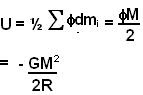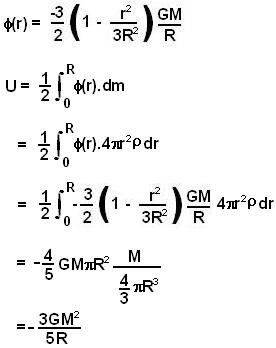## Sunday, September 21, 2008

### Irodov Problem 1.217a)
Imagine that the shell is made of a large number of very tiny particle point massessuch that. The total interaction energy of the shell is the energy required to separate all these point masses of the spherical shell against their mutual attractive forces into infinity. Let E(dmi,dmj) be work done by gravity when masses dmi and dmj are pulled apart to infinity - this is the interaction energy of two particles dmi and dmj. The the total interaction energy of the entire spherical shell is given by the sum of the interaction energies of all pairs of particles E(dm1,dm2) + E(dm1,dm3) + E(dm2,dm3) + E(dm1,dm4) + E(dm2,dm4) + E(dm3,dm4) + ...

The gravitational field strength at any point outside the spherical shell at a distance r from its center can be determined using Gauss law of gravity and choosing a spherical Gaussian surface of radius r and that is concentric with the spherical shell as shown in the figure. So we have,Now we can determine the gravitational potential at any given point on the spherical shell as,The potential energy of any point mass dm in the spherical shell will be. This gravitaional potential energy is actually the work done by gravity in pulling this point mass dm away from the rest of the sphere to infinity.

In other words,= E(dm1,dm2) + E(dm1,dm3)+ E(dm1,dm4).... or,Now since E(dm1,dm2) = E(dm2,dm1) in the sumwe end up adding the gravitational interaction energy of each pair of point masses dmi,dmj , E(dmi,dmj), twice. In other words the total energy of interaction of all the particles in the spherical shell is given by,b)
The basic idea behind the solution is identical to that in part a) execpt that now we have consider the entire volume of the sphere. The gravitational potential at a point that is r distance away from the center of the sphere was computed in the solution to problem 1.217 as,As in case of part a), the total gravitational energy of interaction is given by,